# Convert From Unix Epoch to the Date in PostgreSQL

PostgreSQL offers numerous date and time functions for users. These functions and operators are specially designed to help the user with dynamic calculations.

How to convert Unix Timestamp to Da...
How to convert Unix Timestamp to DateTime using Apex in Salesforce?

Date and time functions are one of the most used functions in software and applications.

For instance, a simple age calculation of the user requires a date and time function at the back end to perform all the required calculations.

The current date is compared with the user’s birth date to calculate the age. Several other applications require the use of date and time functions and operators.

This article has been specially curated to help you calculate the Unix `epoch` date.

## What Is Unix `Epoch`

Before moving on with the article, it is necessary to clarify what Unix `Epoch` is. The Unix time is a system in computing that is used to describe a point in time.

It’s the number of seconds that have passed since the Unix `Epoch`. However, this time system does not count leap seconds.

It returns the numbers of seconds, excluding leap seconds that have elapsed since the Unix `Epoch`.

The question remains, what is the Unix `Epoch`? Unix `Epoch` is a fixed date and time: January 1st, 1970, at 00:00:00 UTC.

The engineers arbitrarily set the Unix `Epoch` to coordinate and set a uniform date for the start of time. In other words, it helps to coordinate the date and time across the world since the number of seconds elapsed from the Unix `Epoch` date remains the same for a particular time worldwide.

## Convert From Unix `Epoch` to the Date in PostgreSQL

There are numerous methods to get the current date in PostgreSQL. Before moving on to the SQL statement and function used to convert the Unix `Epoch` to the current date, the following describes a few necessary SQL statements in detail.

### How to Get the Current Time

If you want to get the date from the Unix `Epoch`, it is essential to understand how to get the current time in PostgreSQL. The current time can then be used to calculate the date.

The following statement is used in PostgreSQL to get the current time.

``````SELECT now();
``````

The function `now()` is used in PostgreSQL to get the current time. It returns the timestamp, which is used to calculate the date later.

The result of the following query is as follows: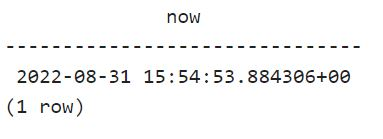## `Epoch` From Current Time

Unix `Epoch` has been described in the section above in detail. The following statement can be used to calculate the number of seconds elapsed from Unix `Epoch`.

``````SELECT EXTRACT(epoch from now());
``````

The SQL query can be executed in PostgreSQL to get the number of seconds passed since the Unix `Epoch`. The `now()` function has been used to get the current time along with the `epoch` in the SQL statement.

The result of the following query is as follows: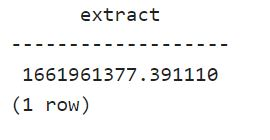## Get Date and Time From `Epoch`

The `EXTRACT` SQL statement, along with `epoch` and `now()`, returns the number of seconds elapsed from the Unix `Epoch`. However, what should be done if you want to get the date and time from the `Epoch`?

You can use two methods to get the date and time from the `Epoch`.

1. The `to_timestamp()` function
2. The timestamp with the time zone approach

Both of these procedures are described below.

## the `to_timestamp()` Function

The `to_timestamp()` function can be used along with `epoch` and `now()` to get the date and time from the Unix `Epoch`.

The `to_timestamp()` function takes a double precision argument. It converts Unix `Epoch` to timestamp with timezone.

### Get Date and Time Using the `to_timestamp()` Function

The following statement can be executed in PostgreSQL to get the date and time from the `Epoch`.

``````SELECT to_timestamp(extract(epoch from now()));
``````

The query result is as follows: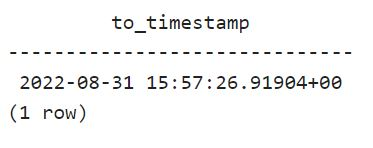### Get Date From `Epoch` Using the `to_timestamp()` Function

If you are interested in only getting the date from the `Epoch`, you can use the `to_timestamp()` function with a slightly different approach. The following statement depicts how to get the date from the epoch using the `to_timestamp()` function.

``````SELECT to_timestamp(extract(epoch from now()))::date;
``````

This query only returns the date from the Unix `epoch`. The result of the query is below.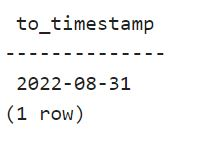## the Timestamp With Time Zone Approach

The second approach that can be used to convert the Unix `Epoch` to date or time is using the `"timestamp with time zone"` approach. It performs the same function as the `to_timestamp()` function.

This approach is an alternative to the `to_timestamp()` function. If one approach does not work, you can follow the other approach to get the work done.

### Get Date and Time Using Timestamp Approach

The following statement can be executed to get the date and time from Unix `Epoch`.

``````SELECT timestamp with time zone 'epoch' + extract(epoch from now()) * interval '1 second';
``````

This approach adds seconds to the `epoch`. It returns the date and time from the Unix `Epoch`.

The result of the query is as follows: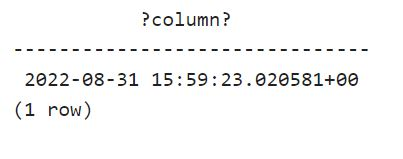### Get Date From `Epoch` Using Timestamp Approach

If you are interested in only getting the date from the `Epoch`, the timestamp approach can be altered slightly differently to receive the required output.

The following statement performs the task.

``````SELECT (timestamp with time zone 'epoch' + extract(epoch from now()) * interval '1 second') :: date;
``````

The statement returns only the date from the `Epoch`. Therefore, this statement can be used if you do not want the time along with the date.

Or, as mentioned in the section above, a similar statement with the `to_timestamp()` function can be used.

The result of the query is as follows: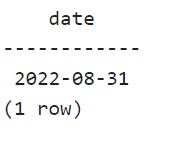## Conclusion

Numerous date and time functions in PostgreSQL help the user with different calculations.

The `to_timestamp()` function and the `"timestamp with time zone"` approach were used for this article to get the date and time from the epoch. Every step has been explained in detail for the user to follow.

## Related Article - PostgreSQL Timestamp

• Insert Current Timestamp in PostgreSQL